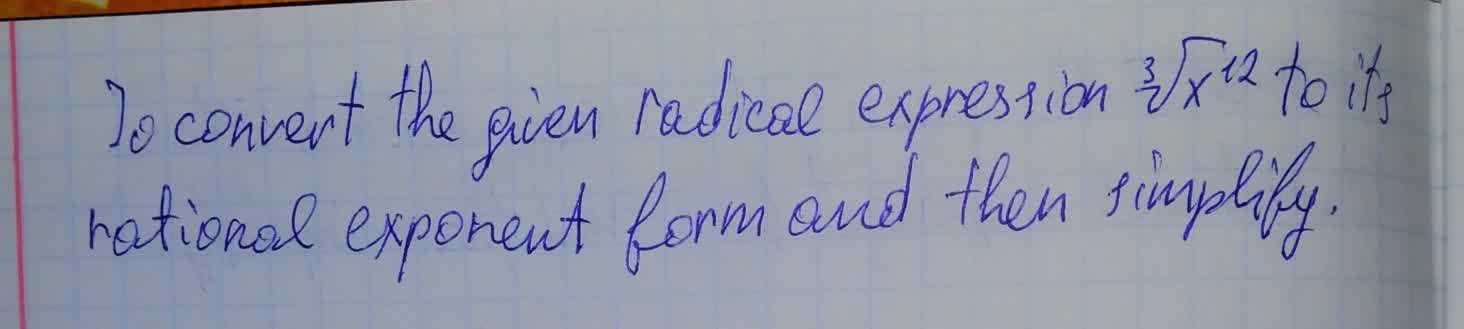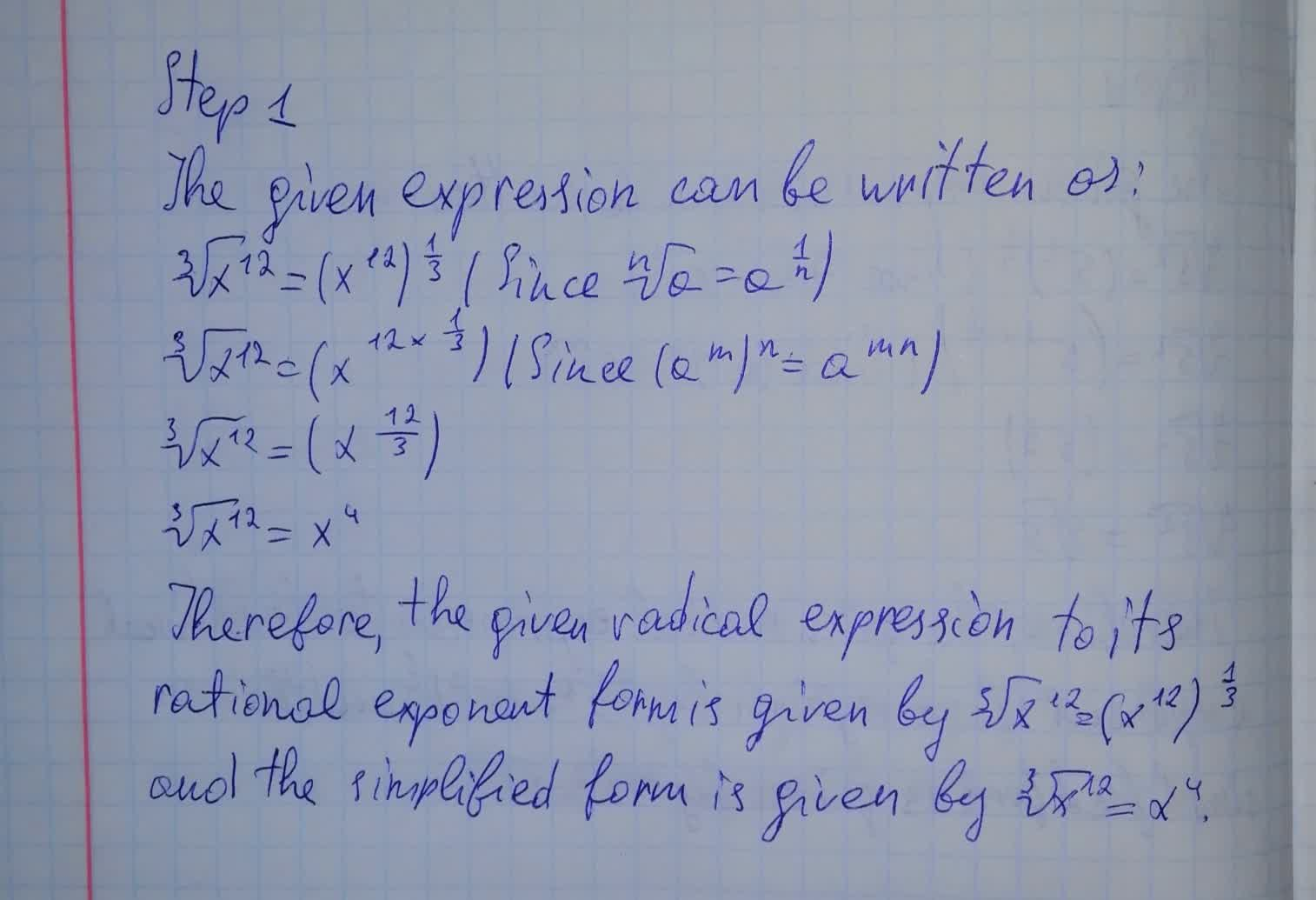To convert the given radical expression \sqrt{x^{12}} to its rational exponent form and then simplifyJason Farmer 2021-08-08 Answered

To convert the given radical expression $$\sqrt{x^{12}}$$ to its rational exponent form and then simplify• Questions are typically answered in as fast as 30 minutes

Solve your problem for the price of one coffee

• Math expert for every subject
• Pay only if we can solve itrogreenhoxa8
Now we haveNot exactly what you’re looking for?Vasquez

Step 1

The given expression can be written as:

$$\sqrt{x^{12}}=(x^{12})^{\frac 13} \ \ \ \ (\text{ Since } \sqrt[n]{a}=a^{\frac 1n})$$

$$\sqrt{x^{12}}=(x^{12 \times \frac 13}) \ \ \ (\text{ Since } (a^m)^n=a^{mn})$$

$$\sqrt{x^{12}}=(x^{\frac{12}{3}})$$

$$\sqrt{x^{12}}=x^4$$

Therefore, the given radical expression to its rational exponent form is given by $$\sqrt{x^{12}}=(x^{12})^{\frac 13}$$ and the simplifiend form is given by $$\sqrt{x^{12}}=x^4$$